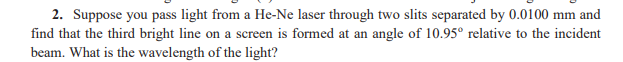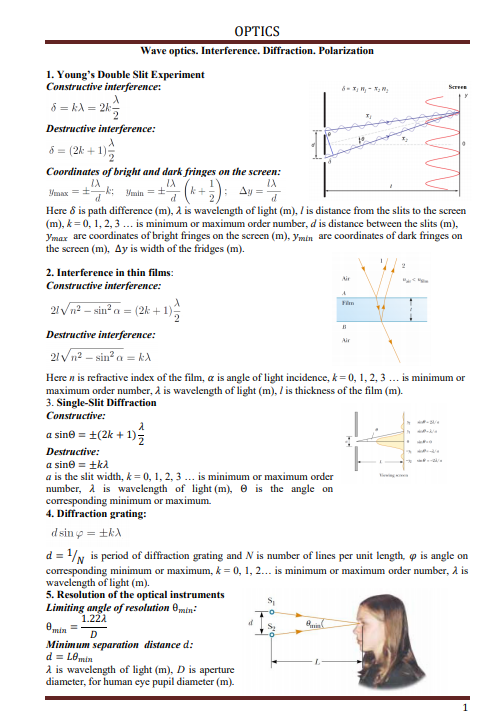# 2. Suppose you pass light from a He-Ne laser through two slits separated by 0.0100 mm andfind that the third bright line on a screen is formed at an angle of 10.95° relative to the incidentbeam. What is the wavelength of the light? OPTICSWave optics. Interference. Diffraction. Polarization1. Young's Double Slit ExperimentConstructive interference:Sereen6 = kA = 2kDestructive interference:8 = (2k + 1);Coordinates of bright and dark fringes on the screen:IA(*+): av=-k: VanimMmax =+Here 8 is path difference (m), a is wavelength of light (m), / is distance from the slits to the screen(m), k = 0, 1, 2, 3 ... is minimum or maximum order number, d is distance between the slits (m),Ymax are coordinates of bright fringes on the screen (m), ymin are coordinates of dark fringes onthe screen (m), Ay is width of the fridges (m).2. Interference in thin films:AirConstructive interference:21Vn? – sin? a =-= (2k +1)вDestructive interference:Air21Vn2 – sin² a = kAHere n is refractive index of the film, a is angle of light incidence, k= 0, 1, 2, 3 ... is minimum ormaximum order number, å is wavelength of light (m), / is thickness of the film (m).3. Single-Slit DiffractionConstructive:a sine = +(2k + 1)5Destructive:a sine = tkda is the slit width, k = 0, 1, 2, 3 ... is minimum or maximum ordernumber, À is wavelength of light (m), e is the angle oncorresponding minimum or maximum.4. Diffraction grating:d sin g = ±kAd = /N is period of diffraction grating and N is number of lines per unit length, o is angle oncorresponding minimum or maximum, k = 0, 1, 2... is minimum or maximum order number, å iswavelength of light (m).5. Resolution of the optical instrumentsLimiting angle of resolution emin:1.222e,minMinimum separation distance d:d = L0minA is wavelength of light (m), D is aperturediameter, for human eye pupil diameter (m).

Question
45 viewshelp_outlineImage Transcriptionclose2. Suppose you pass light from a He-Ne laser through two slits separated by 0.0100 mm and find that the third bright line on a screen is formed at an angle of 10.95° relative to the incident beam. What is the wavelength of the light? fullscreenhelp_outlineImage TranscriptioncloseOPTICS Wave optics. Interference. Diffraction. Polarization 1. Young's Double Slit Experiment Constructive interference: Sereen 6 = kA = 2k Destructive interference: 8 = (2k + 1); Coordinates of bright and dark fringes on the screen: IA (*+): av= -k: Vanim Mmax =+ Here 8 is path difference (m), a is wavelength of light (m), / is distance from the slits to the screen (m), k = 0, 1, 2, 3 ... is minimum or maximum order number, d is distance between the slits (m), Ymax are coordinates of bright fringes on the screen (m), ymin are coordinates of dark fringes on the screen (m), Ay is width of the fridges (m). 2. Interference in thin films: Air Constructive interference: 21Vn? – sin? a =- = (2k +1) в Destructive interference: Air 21Vn2 – sin² a = kA Here n is refractive index of the film, a is angle of light incidence, k= 0, 1, 2, 3 ... is minimum or maximum order number, å is wavelength of light (m), / is thickness of the film (m). 3. Single-Slit Diffraction Constructive: a sine = +(2k + 1)5 Destructive: a sine = tkd a is the slit width, k = 0, 1, 2, 3 ... is minimum or maximum order number, À is wavelength of light (m), e is the angle on corresponding minimum or maximum. 4. Diffraction grating: d sin g = ±kA d = /N is period of diffraction grating and N is number of lines per unit length, o is angle on corresponding minimum or maximum, k = 0, 1, 2... is minimum or maximum order number, å is wavelength of light (m). 5. Resolution of the optical instruments Limiting angle of resolution emin: 1.222 e, min Minimum separation distance d: d = L0min A is wavelength of light (m), D is aperture diameter, for human eye pupil diameter (m). fullscreen
check_circle

star
star
star
star
star
1 Rating
Step 1

According to the interference phenomenon, the wavelength of the light is,

Step 2

Substitute the ...

### Want to see the full answer?

See Solution

#### Want to see this answer and more?

Solutions are written by subject experts who are available 24/7. Questions are typically answered within 1 hour.*

See Solution
*Response times may vary by subject and question.
Tagged in

### Ray Optics